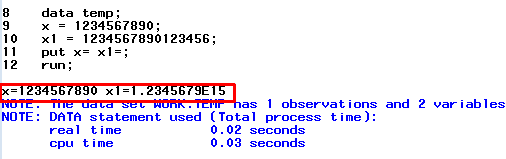# SAS : Length of Numeric Variables

This tutorial describes how SAS treats length of numeric variables in data sets. It is often asked in interviews if default length of numeric variable is 8, how would you store a numeric variable having value more than 8 digits (for example, 123456789). It seems to be a simple question but confusing. Hence, it is required to pay attention how SAS stores numeric variables.

Solution :

In SAS, the default length of a numeric variable is 8 bytes. Pay attention to bytes. The limit is NOT 8 digits but 8 bytes. 8 bytes means we can store up to 16 digits for a numeric variable in SAS. In other words, the default length of numeric variable is 16 digits. It is important to note that the minimum length of a numeric is 3 bytes. It does not mean it cannot store a numeric value lower than 3 digits. It can store values of 1 or 2 digits. See the table below what bytes mean in terms of digits.

Length (Bytes) Largest Numeric Value
3 8192
4 2097152
5 536870912
6 137438953472
7 35184372088832
8 9007199254740992

The length of a numeric variable lies between 3 and 8 bytes. It means SAS can store a numeric value from 1 to 16 digits.

See the example below -

Run the following program and see log. It would give you how SAS keeps numeric values.
data temp;
x = 1234567890;
x1 = 1234567890123456;
put x= x1=;
run;SAS : Length of Numeric Variable
If you look at the image above, SAS stores variables x and x1 without any issue. But the format of the variable x1 is in E notation. See how it works -
1.23456789E15 is equivalent to 1.23456789 𝗑 10¹⁵
Rule -

If the the value of numeric variable is less than or equal to 12 digits it is displayed normally which means the format of the numeric value does not change to E notation. If it is more than 12 digits, the format changes to E notation. To avoid E notation, we can use best16. format which prevents to change the format of the larger values.
data temp;
x = 1234567890;
x1 = 1234567890123456;
format x1 best16.;
put x= x1=;
run;
Related Posts
ShareDeepanshu founded ListenData with a simple objective - Make analytics easy to understand and follow. He has over 10 years of experience in data science. During his tenure, he worked with global clients in various domains like Banking, Insurance, Private Equity, Telecom and HR.

2 Responses to "SAS : Length of Numeric Variables"
1.So can we describe for example 'BEST16' as also being 16 significant figures?

2.how to restrict numeric values length , as we just want correct telephone numbers digit should be equal to 10.

Next → ← Prev